# Managerial Economics Study Set 8

## Quiz 9 : Applications of Cost TheoryStudy FlashcardsLooking for Economics Homework Help?

## Quiz 9 :Applications of Cost Theory

Question TypeIn making an entry/exit decision, if competitive pressure is projected to force the price down to $300, what is the break-even unit sales volume this company should have projected as part of its business plan before entering this market and should reconsider each time it considers leaving (exiting) this business altogether Free Essay Answer: Answer: Following table lists the cost of flying a charter flight from Baltimore to Las Vegas (and return next day) on a seven year old Boeing 737-8000 with 120 occupied seats.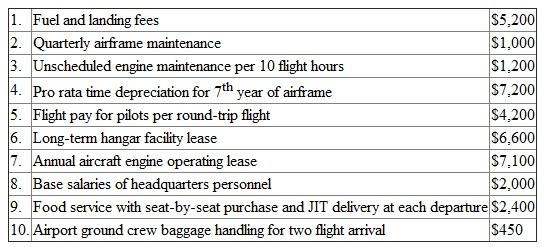The break-point occurs when total revenue equals total costs. Break-even unit sales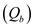are calculated as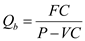…… (1) Where, FC are the fixed cost for the round-trip, P is the price per seat and VC are the variable cost for a round trip. In case of charter flight, variable cost would vary with the number of occupied seat, that is, food service with seat-by-seat purchase and JIT delivery at each departure. The number of seats occupied is 120 and the variable cost for 120 passengers is$2,400.
Thus, the variable cost of sending one more person aboard a charter flight is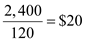each way, or $40 for a round-trip. All the costs except food service are fixed costs. Thus, the fixed cost of a round-trip is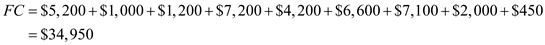Substitute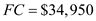,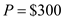and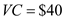in (1)Thus, the break-even unit sales are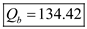. Tags Choose question tagWhat type of cost-output relationship (linear, quadratic, cubic) is suggested by these statistical results Free Essay Answer: Answer: The following regression equation is constructed on the basis of the statistical data on 109 selected high schools:Here,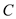is the operating expenditure per student,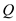is the students' average daily attendance,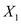is the average teacher salary,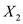is the number of credit units offered,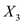is the average number of courses taught per teacher,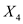is the change in enrollment between 1957 and 1960, and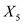is the percentage of classrooms built after 1950. An asterisk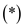over t-values in parenthesis indicates that the result is statistically significant at 0.01 level. It is evident from the information given that the term is statistically significant at 0.01 level. Thus, a quadratic average cost-output relationship, that is, a cubic total cost-output relationship is suggested by these statistical results. Tags Choose question tagMcKee Corporation has annual fixed costs of$12 million. Its variable cost ratio is.60. a. Determine the company's break-even dollar sales volume. b. Determine the dollar sales volume required to earn a target profit of $3 million. Free Essay Answer: Answer: a) Company's breakeven dollar sales volume. McKee Corporation has annual fixed cost of$12,000,000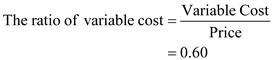The ratio of variable cost is 0.60; hence, the price is $1.Required amount is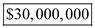. b) Sale volume required to earn the target profit of$3 million.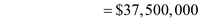Target volume is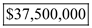.

Tags
Choose question tagWhat are the variable costs for the decision to send one more person aboard a charter flight that is already 80 percent booked
Essay
Tags
Choose question tagA study of the costs of electricity generation for a sample of 56 British firms in 1946-1947 yielded the following long-run cost function: 16 AVC = 1.24 +.0033Q +.0000029Q 2 .000046QZ .026Z +.00018Z 2 where AVC = average variable cost (i.e., working costs of generation), measured in pence per kilowatt-hour (kWh). (A pence was a British monetary unit equal, at that time, to 2 cents U.S.) Q = output; measured in millions of kWh per year Z = plant size; measured in thousands of kilowatts a. Determine the long-run variable cost function for electricity generation. b. Determine the long-run marginal cost function for electricity generation. c. Holding plant size constant at 150,000 kilowatts, determine the short-run average variable cost and marginal cost functions for electricity generation. d. For a plant size equal to 150,000 kilowatts, determine the output level that minimizes short-run average variable costs. e. Determine the short-run average variable cost and marginal cost at the output level obtained in Part (d). 16 Johnston, Statistical Cost Analysis, Chapter 4, op. cit.
Essay
Tags
Choose question tagA study of 86 savings and loan associations in six northwestern states yielded the following cost function: 15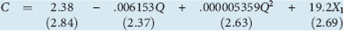where C = average operating expense ratio, expressed as a percentage and defined as total operating expense ($million) divided by total assets ($ million) times 100 percent Q = output; measured by total assets ($million) X 1 = ratio of the number of branches to total assets ($million) Note: The number in parentheses below each coefficient is its respective t-statistic. a. Which variable(s) is(are) statistically significant in explaining variations in the average operating expense ratio b. What type of cost-output relationship (e.g., linear, quadratic, or cubic) is suggested by these statistical results c. Based on these results, what can we conclude about the existence of economies or diseconomies of scale in savings and loan associations in the Northwest 15 Holton Wilson, "A Note on Scale Economies in the Savings and Loan Industry," Business Economics (January 1981), pp. 45-49.
Essay
Tags
Choose question tagBased on the results of this study, what can we conclude about the existence of economies or diseconomies in operating a public high school
Essay
Tags
Choose question tagBased on the scatter diagram in Question, what kind of mathematical relationship would appear to exist between enrollment and operating expenditures per student In other words, do operating expenditures per student appear to (i) be constant (and independent of enrollment), (ii) follow a linear relationship as enrollment increases, or (iii) follow some sort of nonlinear U-shape (possibly quadratic) relationship as enrollment increases As part of this study, the following cost function was developed: C = f(Q, X 1 , X 2 , X 3 , X 4 , X 5 ) where C = operating expenditures per student in average daily attendance (measured in dollars) Q = enrollment (number of students in average daily attendance) X 1 = average teacher salary X 2 = number of credit units ("courses") offered X 3 = average number of courses taught per teacher X 4 = change in enrollment between 1957 and 1960 X 5 = percentage of classrooms built after 1950 Variables X 1 , X 2 , and X 3 were considered measures of teacher qualifications, breadth of curriculum, and the degree of specialization in instruction, respectively. Variable X 4 measured changes in demand for school services that could cause some lagging adjustments in cost. Variable X 5 was used to reflect any differentials in the costs of maintenance and operation due to the varying ages of school properties. Statistical data on 109 selected high schools yielded the following regression equation: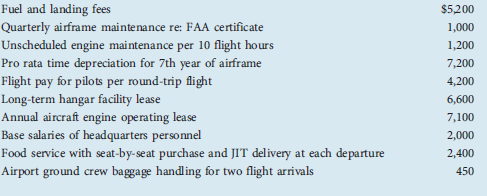Notes: The numbers in parentheses are the t-scores of each of the respective (b) coefficients. An asterisk (*) indicates that the result is statistically significant at the 0.01 level. Question Plot the data in columns B and C in an output (enrollment-) cost graph and sketch a smooth curve that would appear to provide a good fit to the data.
Essay
Tags
Choose question tagHolding constant the effects of the other variables (X 1 through X 5 ), determine the enrollment level (Q) at which average operating expenditures per student are minimized. (Hint: Find the value of Q that minimizes the ( C/ Q function.)
Essay
Tags
Choose question tagCharter contracts are negotiable, and charter carriers receive many contract offers that do not promise $300 prices or 80-percent-full planes. Should the airline accept a charter flight proposal from a group that offers to guarantee the sale of 90 seats at$250 Why or why not
Essay
Tags
Choose question tagReferring to Exercise again: a. Holding constant the effects of branching (X 1 ), determine the level of total assets that minimizes the average operating expense ratio. b. Determine the average operating expense ratio for a savings and loan association with the level of total assets determined in Part (a) and 1 branch. Same question for 10 branches. Exercise A study of 86 savings and loan associations in six northwestern states yielded the following cost function: 15where C = average operating expense ratio, expressed as a percentage and defined as total operating expense ($million) divided by total assets ($ million) times 100 percent Q = output; measured by total assets ($million) X 1 = ratio of the number of branches to total assets ($million) Note: The number in parentheses below each coefficient is its respective t-statistic. a. Which variable(s) is(are) statistically significant in explaining variations in the average operating expense ratio b. What type of cost-output relationship (e.g., linear, quadratic, or cubic) is suggested by these statistical results c. Based on these results, what can we conclude about the existence of economies or diseconomies of scale in savings and loan associations in the Northwest 15 Holton Wilson, "A Note on Scale Economies in the Savings and Loan Industry," Business Economics (January 1981), pp. 45-49.
Essay
Tags
Choose question tagAssuming that all other factors remain unchanged, determine how a firm's breakeven point is affected by each of the following: a. The firm finds it necessary to reduce the price per unit because of increased foreign competition. b. The firm's direct labor costs are increased as the result of a new labor contract. c. The Occupational Safety and Health Administration (OSHA) requires the firm to install new ventilating equipment in its plant. (Assume that this action has no effect on worker productivity.)
Essay
Tags
Choose question tagAgain, holding constant the effects of the other variables, use the C/ Q function to determine, for a school with 500 students, the reduction in per-student operating expenditures that will occur as the result of adding one more student.
Essay
Tags
Choose question tagIf one were trying to decide whether to operate (fly) or not fly an unscheduled round-trip charter flight, what would be the total direct fixed costs and variable costs of the flight
Essay
Tags
Choose question tagWhat variables (other than enrollment) would appear to be most important in explaining variations in operating expenditures per student
Essay
Tags
Choose question tagWhat are the total contributions of the charter flight with 90 seats at \$250 per seat
Essay
Tags
Choose question tagAgain, holding the other variables constant, what would be the saving in perstudent operating expenditures of an increase in enrollment from 500 to 1,000
Essay
Tags
Choose question tagPlot the data in columns B and C in an output (enrollment-) cost graph and sketch a smooth curve that would appear to provide a good fit to the data.
Essay
Tags
Choose question tagIdentify the indirect fixed costs of the charter service for a particular one of many such charters this month.
Essay